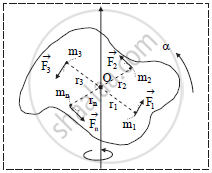HSC Science (General) 11thMaharashtra State Board
Share

Obtain an Expression for Torque Acting on a Rotating Body with Constant Angular Acceleration. Hence State the Dimensions and Si Unit of Torque. - HSC Science (General) 11th - Physics

ConceptTorque and Angular Momentum

Question

Obtain an expression for torque acting on a rotating body with constant angular acceleration. Hence state the dimensions and SI unit of torque.

Solution

a. Suppose a rigid body consists of n particles of masses m1, m2, m3, ......, mn which are situated at distances r1, r2, r3, …, rn respectively, from the axis of rotation as shown in figure.
b. Each particle revolves with angular acceleration alpha.

c. Let F1, F2, F3, …., Fn be the tangential force acting on particles of masses, m1, m2, m3, …, mn respectively.

d. Linear acceleration of particles of masses m1, m2,…, mn are given by, a1 = r1α, a2 = r2α, a3 = r3α, …, an = rnαe. Magnitude of force acting on particle of mass m1 is given by,
F_1=m_1a_1=m_1r_1alpha          [therefore a=r alpha]
Magnitude of torque on particle of mass m1 is given by,
tau_1=F_1r_1 sin theta
But,theta=90^@            [∵ Radius vector is ⊥ar to tangential force

tau_1=F_1r_1sin90^@

=F_1r_1

=m_1a_1r_1

tau_1=m_1r_1^2alpha

similarly

tau_2=m_2r_2^2alpha

tau_3=m_3r_3^2alpha

tau_n=m_nr_n^2alpha

f.   Total torque acting on the body,

tau=tau_1+tau_2+tau_3+..........+tau_n

tau=m_1r_1^2alpha+m_2^2alpha+m_2r_3^2alpha+......+m_nr_n^2alpha

therefore tau=[sum_(i=1)^nm_ir_t^2]alpha

But sum_(i=1)^nm_ir_i^2=I

therefor tau=Ialpha

g. Unit: Nm in SI system.
h. Dimensions: [M^1L^2T^-2]

Is there an error in this question or solution?
Solution Obtain an Expression for Torque Acting on a Rotating Body with Constant Angular Acceleration. Hence State the Dimensions and Si Unit of Torque. Concept: Torque and Angular Momentum.
S